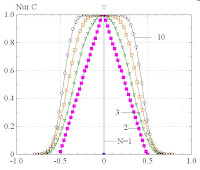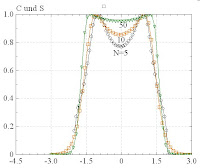## Wednesday, December 17, 2008

###  Finite Size PDF of independent points in 1D

The following relates to posts  and :

--------------------------------------------------------------------------------

Simplifications:

In order to indentify the minimum conditions of a double-peaked universal PDF, the situation can be simplified in several aspects:

A) 1D space:

By restricting the trajectories to one spatial dimension x, rotations are not required any longer.

B) Sets of N independent points:

Trajectories are normally generated by subsequently adding random increments (steps) to the respective last position of a walker. Here, in order to avoid any correlations between successive points, the N positions in each point set are drawn independently from a fixed probability density.

C) Random points equally distributed in [0,1]:

Let this probability density be constant in the intervall [0,1] and zero outside.

--------------------------------------------------------------------------------

Statistical quantities of point sets:

For each point set {x_i}, the center of mass is computed,
\overline{x} = \left\langle x_i \right\rangle_i = \frac{1}{N}\sum_{i=1}^N x_i \;,

as well as the standard deviation
\sigma = \sqrt{\left\langle (x_i-\overline{x})^2\right\rangle_i} \;.
--------------------------------------------------------------------------------

Transformations on point sets:

C-Operation (Center):
x_i \rightarrow (x_i-\overline{x}) \;\;\forall i

S-Operation (Scale):
x_i \rightarrow \overline{x}+(x_i-\overline{x})/\sigma \;\;\forall i

Note that C and S commute with each other.

--------------------------------------------------------------------------------

Effects of transformations:

The effects of C and S on the resulting averaged distributions P(x) are discussed in the following:

* No C, no S:

Direct averaging, without any C or S, yields the expected box-shaped distribution in [0,1], centered around 1/2.

* Only C:

Applying only the C-operation yields PDFs centered around zero. For N=1 one obtains a delta-function, for N=2 a triangular function, etc. For very large N, the box is recovered:* Only S:

Scaling alone produces already a double-peak structure, centered around 1/2. However, it is a finite size effect that quickly disappears for long trajectories:* C and S:

The combined effect of C and S yields a double-peak centered around zero:If instead of the box-shaped distribution, a Gaussian distribution is used for the random points, the results are qualitatively the same. However, the double peak is much weaker pronounced and visible only for very small trajectory lengths.

--------------------------------------------------------------------------------

Summary:

Summing up, the double-peak is not a very remarkable feature of 1D trajectories.

--------------------------------------------------------------------------------

Origin of double-peak:

Take the extreme case of N=2: After a C-operation the two points lie symmetrically left and right from x=0. An additional S-operation scales the distance between the two points to the norm value, and the average distribution P(x) consequently consists of two delta-functions.

On the other hand, when N becomes very large, the histogram of each individual trajectory will already reflect the ensemble average very closely. The CS-operations therefore do not change the shape of this distribution qualitatively and one expects for P(x) to recover the fundamental distribution (in our case: box-shape).

Then, for intermediate lengths N, one expects a gradual interpolation between the double-delta-peak and a box-distribution.

--------------------------------------------------------------------------------